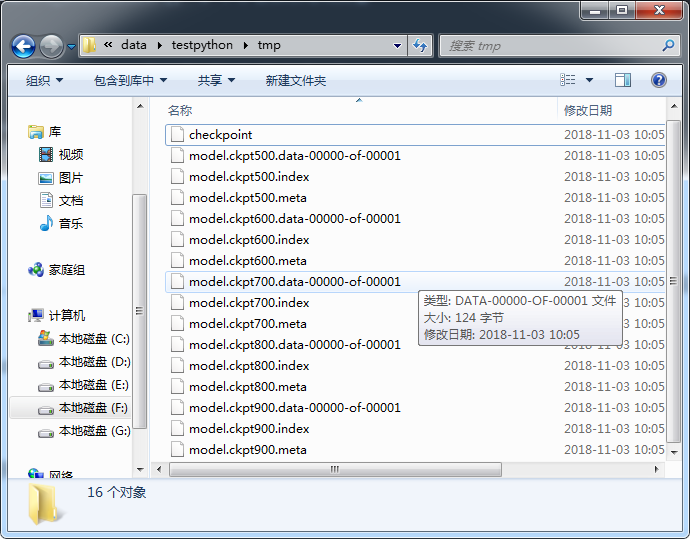TensorFlow模型的儲存與恢復（使用tf.train.Saver()和saver.restore()）：

首先我們需要建立一個用來儲存模型的物件saver：

saver = tf.train.Saver(max_to_keep=3)

如果我們想在每次迴圈結束時儲存一個最新生成的模型，可以這樣寫：

import tensorflow as tf

saver=tf.train.Saver()
with tf.Session() as sess:
sess.run(tf.global_variables_initializer())
for i in range(iteration):
# 這裡代表我們進行的訓練過程
.....
# 訓練完後我們要儲存模型
saver.save(sess, save_path, global_step=i)
# save_path是儲存模型的路徑，注意儲存路徑要寫上要儲存的檔案的名字，不要寫檔案字尾名

在進行一次 saver.save() 後會建立後3個數據檔案並建立一個檢查點（checkpoint）檔案：恢復模型並繼續訓練或測試：

import tensorflow as tf
import os

saver=tf.train.Saver()
with tf.Session() as sess:
sess.run(tf.global_variables_initializer())
for i in range(iteration):
if os.path.exists("./tmp/checkpoint"):
# 判斷最新的儲存模型檢查點是否存在，如果存在則從最近的檢查點恢復模型
saver.restore(sess, tf.train.latest_checkpoint('./tmp/'))
# 我們也可以取判斷某一個我們儲存的模型是否存在，而不一定是最近的檢查點,如：
# if os.path.exists("./tmp/train_model"):
#     saver.restore(sess,"./tmp/train_model")
# 這裡代表我們進行的訓練過程
.....
# 訓練完後我們要儲存模型
saver.save(sess, save_path, global_step=i)
# save_path是儲存模型的路徑，注意儲存路徑要寫上要儲存的檔案的名字，不要寫檔案字尾名
# global_step=i將儲存的模型的迭代次數作為字尾加入到模型名字中

注意：

saver 的操作必須在 sess 建立後進行，因為saver的方法是tensorflow中的方法，必須要開始Session會話後才能進行。

global_step=i將儲存的模型的迭代次數作為字尾加入到模型名字中。

在測試時，我們可以通過下面的程式碼直接通過meta graph構建網路、載入訓練時得到的引數，並使用預設的session：

saver = tf.train.import_meta_graph(‘model/model.meta’)
saver.restore(tf.get_default_session(),’ model/model.ckpt-16000’)

儲存和讀取模型的實際例子：

import tensorflow as tf
import numpy as np
import os

# 用numpy產生資料x和y
x_data = np.linspace(-1, 1, 300)[:, np.newaxis]  # 轉置
noise = np.random.normal(0, 0.05, x_data.shape)
y_data = np.square(x_data) - 0.5 + noise

# 佔位符x_ph和y_ph
x_ph = tf.placeholder(tf.float32, [None, 1])
y_ph = tf.placeholder(tf.float32, [None, 1])

# 隱藏層
w1 = tf.Variable(tf.random_normal([1, 10]))
b1 = tf.Variable(tf.zeros([1, 10]) + 0.1)
wx_plus_b1 = tf.matmul(x_ph, w1) + b1
hidden = tf.nn.relu(wx_plus_b1)

# 輸出層
w2 = tf.Variable(tf.random_normal([10, 1]))
b2 = tf.Variable(tf.zeros([1, 1]) + 0.1)
wx_plus_b2 = tf.matmul(hidden, w2) + b2
y = wx_plus_b2

# loss函式，方差和平均值
loss = tf.reduce_mean(tf.reduce_sum(tf.square(y_ph - y), reduction_indices=))

# 儲存模型物件saver,設為最多儲存5個模型
saver = tf.train.Saver(max_to_keep=5)

# 判斷模型儲存路徑是否存在，不存在就建立（注意這裡路徑只是目錄，沒有檔名）
if not os.path.exists('tmp/'):
os.mkdir('tmp/')

# 初始化
with tf.Session() as sess:
if os.path.exists('tmp/checkpoint'):  # 判斷最近的檢查點模型是否存在
saver.restore(sess, 'tmp/model.ckpt')  # 如果存在就從模型中恢復變數，注意檔名不要加字尾名
else:
init = tf.global_variables_initializer()  # 不存在就初始化變數
sess.run(init)
for i in range(1000):
_, loss_value = sess.run([train_op, loss], feed_dict={x_ph: x_data, y_ph: y_data})
if i % 100 == 0:
save_path = saver.save(sess, 'tmp/model.ckpt'+str(i))
print("迭代次數：%d , 訓練損失：%s" % (i, loss_value))

注意：

model.ckpt 必須存在我們指定的資料夾中，'tmp/model.ckpt' 這裡至少要有一層資料夾，這個資料夾必須要先建立，否則無法儲存。

執行結果如下：